Case Based Questions (MCQ)

Chapter 9 Class 12 Differential Equations
Serial order wise

## Polio drops are delivered to 50k children in a district. The rate at which polio drops are given is directly proportional to the number of children who have not been administered the drops. By the end of 2nd week half the children have been given the polio drops. How many will have been given the drops by the end of 3rd week can be estimated using the solution to the differential equation dy / dx = k(50 - y) where x denotes the number of weeks and y the number of children who have been given the drops.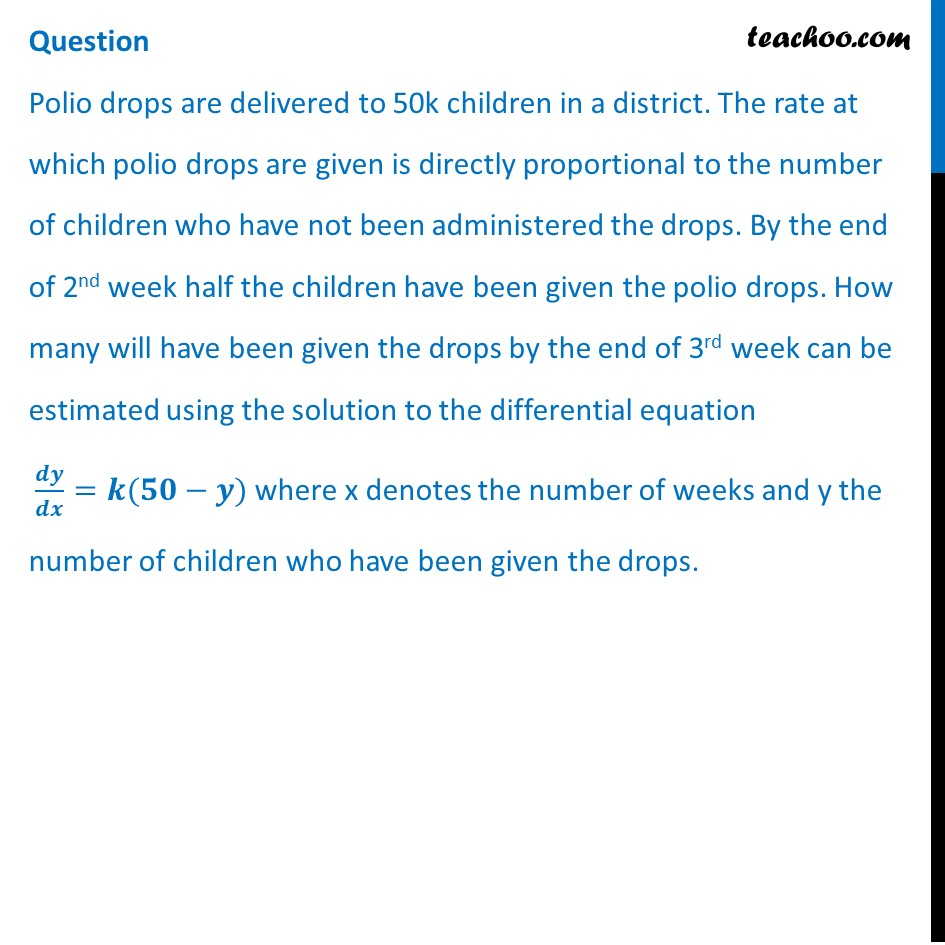## State the order of the above given differential equation.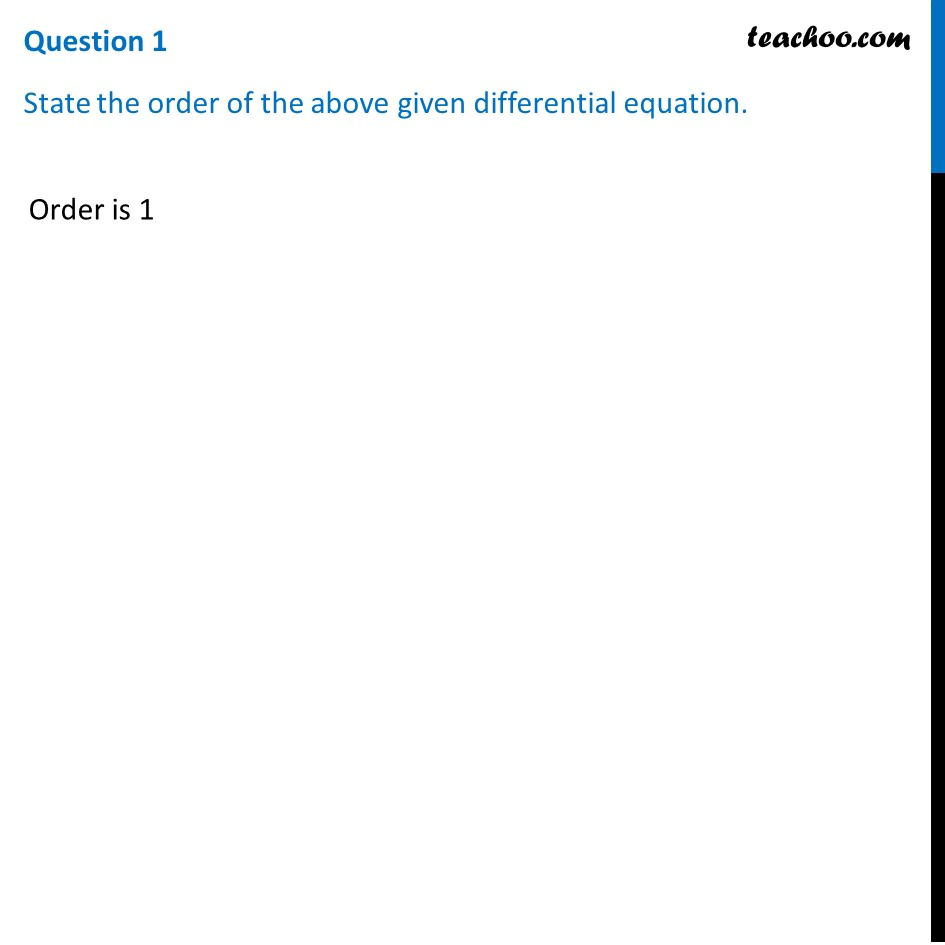## (d) all of the above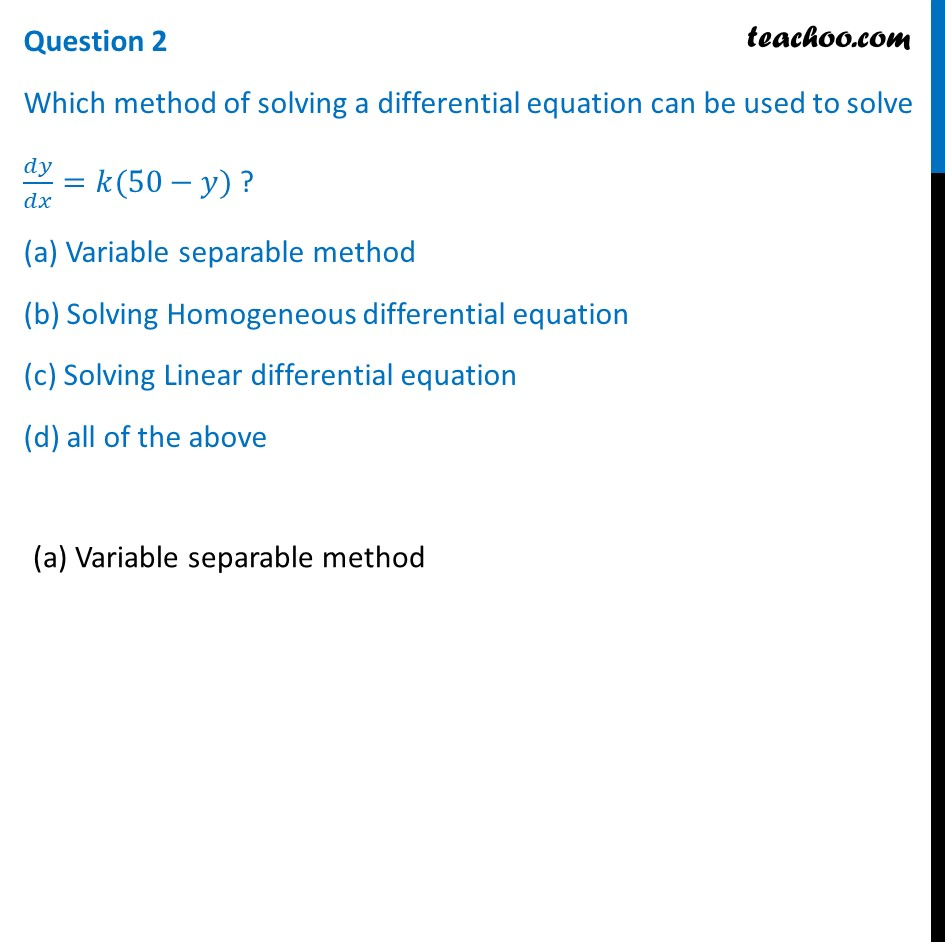## (d) 50-y=kx+ C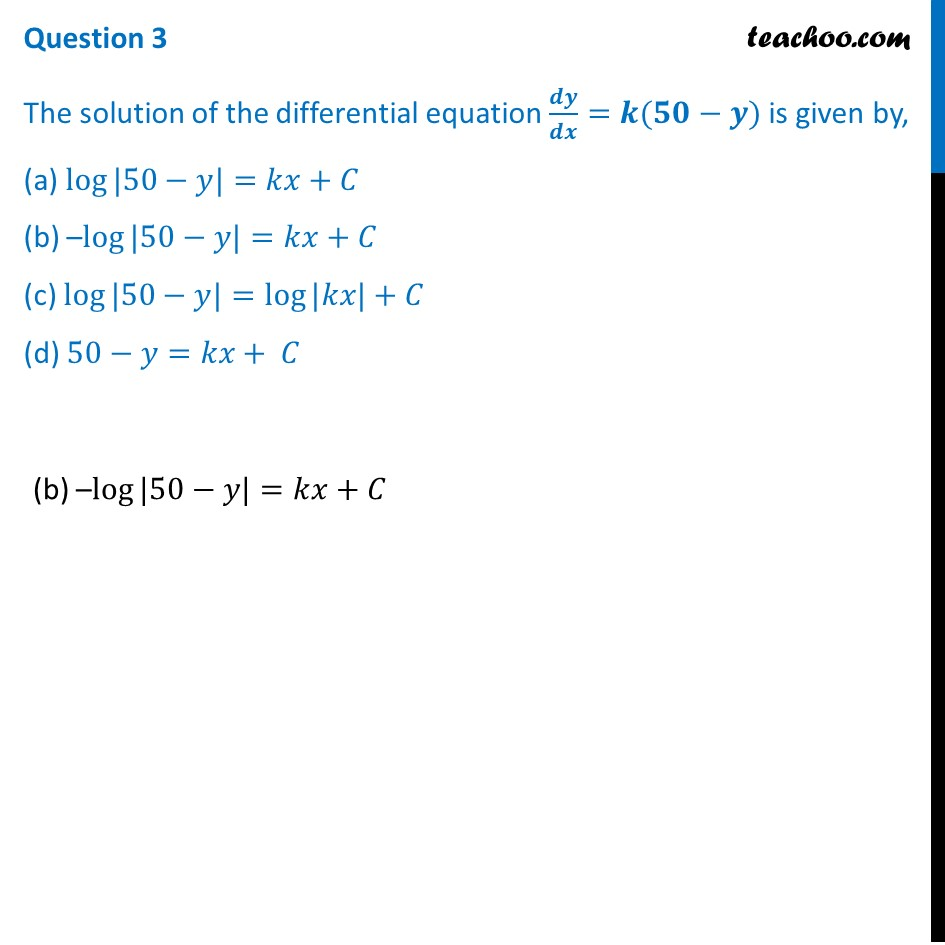Question 4

The value of c in the particular solution given that y(0) = 0 and k = 0.049 is

(a) log 50

(b) log 1/50

(c) 50

(d) –50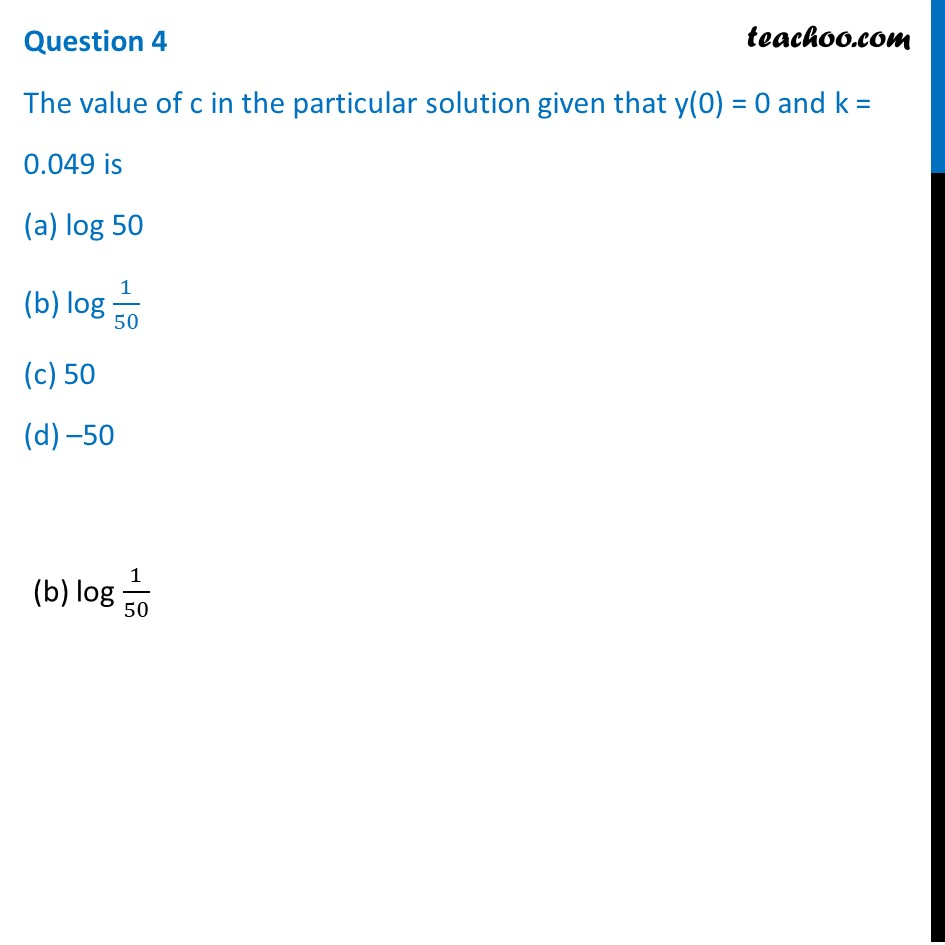## (d) y = 50 (e- kx -1)Introducing your new favourite teacher - Teachoo Black, at only ₹83 per month

### Transcript

Question Polio drops are delivered to 50k children in a district. The rate at which polio drops are given is directly proportional to the number of children who have not been administered the drops. By the end of 2nd week half the children have been given the polio drops. How many will have been given the drops by the end of 3rd week can be estimated using the solution to the differential equation 𝒅𝒚/𝒅𝒙=𝒌(𝟓𝟎−𝒚) where x denotes the number of weeks and y the number of children who have been given the drops. Question 1 State the order of the above given differential equation. Order is 1 Question 2 Which method of solving a differential equation can be used to solve 𝑑𝑦/𝑑𝑥=𝑘(50−𝑦) ? (a) Variable separable method (b) Solving Homogeneous differential equation (c) Solving Linear differential equation (d) all of the above(a) Variable separable method Question 3 The solution of the differential equation 𝒅𝒚/𝒅𝒙=𝒌(𝟓𝟎−𝒚) is given by, (a) log⁡〖|50−𝑦|〗=𝑘𝑥+𝐶 (b) –log⁡〖|50−𝑦|〗=𝑘𝑥+𝐶 (c) log⁡〖|50−𝑦|〗=log⁡〖|𝑘𝑥|〗+𝐶 (d) 50−𝑦=𝑘𝑥+ 𝐶(b) –log⁡〖|50−𝑦|〗=𝑘𝑥+𝐶 Question 4 The value of c in the particular solution given that y(0) = 0 and k = 0.049 is (a) log 50 (b) log 1/50 (c) 50 (d) –50(b) log 1/50 Question 5 Which of the following solutions may be used to find the number of children who have been given the polio drops? (a) y = 50 – 𝑒^𝑘𝑥 (b) y = 50 – 𝑒^(−𝑘𝑥) (c) y = 50 (1−𝑒^(−𝑘𝑥) ) (d) y = 50 (𝑒^(−𝑘𝑥)−1)(c) y = 50 (1−𝑒^(−𝑘𝑥) )